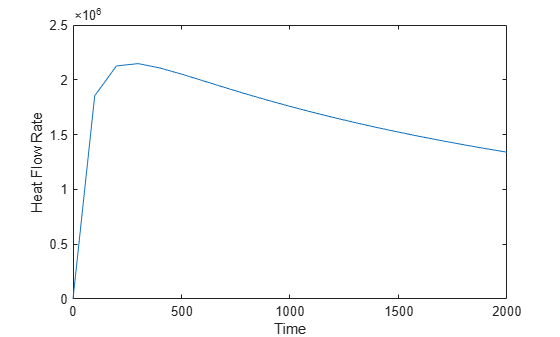# evaluateHeatRate

Evaluate integrated heat flow rate normal to specified boundary

## Syntax

``Qn = evaluateHeatRate(thermalresults,RegionType,RegionID)``

## Description

example

````Qn = evaluateHeatRate(thermalresults,RegionType,RegionID)` returns the integrated heat flow rate normal to the boundary specified by `RegionType` and `RegionID`.```

## Examples

collapse all

Compute the heat flow rate across a face of the block geometry.

Create a steady-state thermal model.

`thermalmodel = createpde("thermal","steadystate");`

Import the block geometry.

```importGeometry(thermalmodel,"Block.stl"); pdegplot(thermalmodel,"FaceLabels","on","FaceAlpha",0.5)```Specify the thermal conductivity of the block.

`thermalProperties(thermalmodel,"ThermalConductivity",80);`

Apply constant temperatures on the opposite ends of the block. All other faces are insulated by default.

```thermalBC(thermalmodel,"Face",1,"Temperature",100); thermalBC(thermalmodel,"Face",3,"Temperature",50);```

Generate mesh.

`generateMesh(thermalmodel,"GeometricOrder","linear");`

Solve the thermal model.

`thermalresults = solve(thermalmodel);`

Compute the heat flow rate across face 3 of the block.

`Qn = evaluateHeatRate(thermalresults,"Face",3)`
```Qn = 4.0000e+04 ```

Compute the heat flow rate across the surface of the cooling sphere.

Create a thermal model for transient analysis.

`thermalmodel = createpde("thermal","transient");`

Create a sphere of radius 1, and assign it to the thermal model.

```gm = multisphere(1); thermalmodel.Geometry = gm;```

Generate mesh.

`generateMesh(thermalmodel,"GeometricOrder","linear");`

Specify thermal properties of the sphere.

```thermalProperties(thermalmodel,"ThermalConductivity",80, ... "SpecificHeat",460, ... "MassDensity",7800);```

Apply a convection boundary condition on the surface of the sphere.

```thermalBC(thermalmodel,"Face",1,... "ConvectionCoefficient",500, ... "AmbientTemperature",30);```

Set the initial temperature.

`thermalIC(thermalmodel,800);`

Solve the thermal model.

```tlist = 0:100:2000; result = solve(thermalmodel,tlist);```

Compute the heat flow rate across the surface of the sphere over time.

```Qn = evaluateHeatRate(result,"Face",1); plot(tlist,Qn) xlabel("Time") ylabel("Heat Flow Rate")```## Input Arguments

collapse all

Solution of a thermal problem, specified as a `SteadyStateThermalResults` object. Create `thermalresults` using the `solve` function.

Example: ```thermalresults = solve(thermalmodel)```

Geometric region type, specified as `"Face"` for 3-D geometry or `"Edge"` for 2-D geometry.

Example: ```Qn = evaluateHeatRate(thermalresults,"Face",3)```

Data Types: `char` | `string`

Geometric region ID, specified as a positive integer. Find the region IDs using the `pdegplot` function with the `"FaceLabels"` (3-D) or `"EdgeLabels"` (2-D) value set to `"on"`.

Example: ```Qn = evaluateHeatRate(thermalresults,"Face",3)```

Data Types: `double`

## Output Arguments

collapse all

Heat flow rate, returned as a real number or, for time-dependent results, a vector of real numbers. This value represents the integrated heat flow rate, measured in energy per unit time, flowing in the direction normal to the boundary. `Qn` is positive if the heat flows out of the domain, and negative if the heat flows into the domain.

## Version History

Introduced in R2017a